# SAT Math Multiple Choice Question 792: Answer and Explanation

### Test Information

Question: 792

12. If w is an integer less than –1, which of the following could be the graph of x + wy = wxy – 3?

• A.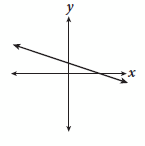• B.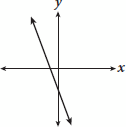• C.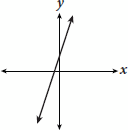• D.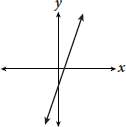Explanation:

C

Difficulty: Hard

Category: Heart of Algebra / Linear Equations

Strategic Advice: When matching a graph to a linear equation, focus on putting the equation in slope-intercept form (y = mx + b) so you can determine what the graph should look like.

Getting to the Answer: Start by collecting the y terms on the left side of the equation and the x terms on the right (don't worry about w yet):

x + wy = wx - y - 3

wy + y = wx - x - 3

Next, factor out x and y, and then divide both sides by the coefficient of y so that it's in slope-intercept form: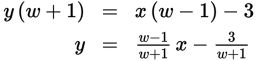The equation represents a line with a slope of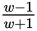and a y-intercept of -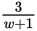. Because you're given that w is an integer less than -1, both the slope and the y-intercept of the line are positive (pick an easy number, such as -2, to plug in for w if you're not certain). This means the line is increasing (going up) from left to right and crosses the vertical axis above 0. The only choice that contains a graph with these characteristics is (C).